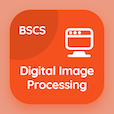Computer Science Courses

Digital Logic Design Exam Prep

Digital Logic Design Practice Test 73

The Book Binary Adder and Subtractor Quiz Questions, binary adder and subtractor MCQ with answers PDF chapter 9-73 to learn online courses, digital logic design tests. Practice MSI and PLD Components trivia questions, binary adder and subtractor Multiple Choice Questions (MCQ Quiz) for online college degrees. The eBook Binary Adder and Subtractor Quiz App Download: binary adder and subtractor, flip-flops in dld, synchronous counters in digital logic design, carry propagation, introduction to digital circuits test prep for associates in computer science.

The Quiz: Addition and subtraction can be combined in one circuit with one common binary is known as PDF, "Binary Adder and Subtractor" App Download (Free) with divider, multiplier, subtractor, and adder choices for top computer science schools. Solve msi and pld components questions and answers, Amazon eBook to download free sample for online degrees.

## Logic Design: Binary Adder & Subtractor Quiz

MCQ: Addition and subtraction can be combined in one circuit with one common binary is known as

A) multiplier
B) divider
C) subtractor

MCQ: JK master-slave flip-flop has Integrated circuit number

A) 7476
B) 7451
C) 7456
D) 7436

MCQ: BCD counter counts from

A) 0 to 5
B) 1 to 5
C) 0 to 9
D) 1 to 9

MCQ: The problem of carrying propagation delay was resolved by

MCQ: Bipolar transistors work on

A) electrons
B) poles
C) neutrons
D) both a and b

### Mock Tests: Digital Logic Design Course Prep

Download Digital Logic Design Quiz App, Digital Image Processing MCQs App and DBMS MCQ App for Android & iOS devices. These Apps include complete analytics of real time attempts with interactive assessments. Download Play Store & App Store Apps & Enjoy 100% functionality with subscriptions!